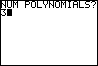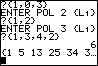# FOIL

## Overview

Multiplies multiple polynomials of any degree. (FOIL - First Outside Inside Last)

## InstructionsEnter the number of polynomials that you wish to multiply. The program can handle polynomials until the calculator runs out of memory. In the example illustrated here, three polynomials are multiplied. The polynomials are entered as lists. In polynomial form the product would be (x^2+3)(x+2)(x^4+3x^3+4x+2). From the program the result is (x^6+5x^5+13x^4+25x^34x^2+30x+12) The 6 that is above the answer list is degree of the polynomial.